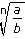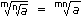# Aptitude - Surds and Indices

### Exercise :: Surds and Indices - Important Formulas

1. Laws of Indices:

1. am x an = am + n

2.  am = am - n an

3. (am)n = amn

4. (ab)n = anbn

5.an = an b bn

6. a0 = 1

2. Surds:

Let a be rational number and n be a positive integer such that a(1/n) = a

Then, a is called a surd of order n.

3. Laws of Surds:

1. a = a(1/n)

2. ab = a x b

3.= a b

4. (a)n = a

5.6. (a)m = am